Courses

# Match the Following, Integer Value of The p-Block Elements , Past Year Questions JEE Advance JEE Notes | EduRev

## JEE : Match the Following, Integer Value of The p-Block Elements , Past Year Questions JEE Advance JEE Notes | EduRev

The document Match the Following, Integer Value of The p-Block Elements , Past Year Questions JEE Advance JEE Notes | EduRev is a part of the JEE Course Class 12 Chemistry 35 Years JEE Mains &Advance Past yr Paper.
All you need of JEE at this link: JEE

Match the Following

DIRECTIONS (Q. 1 to 3) : Each question contains statements given in two columns, which have to be matched. The statements in Column-I are labelled A, B, C and D, while the statements in Column-II are labelled p, q, r, s and t. Any given statement in Column-I can have correct matching with ONE OR MORE statement(s) in Column-II. The appropriate bubbles corresponding to the answers to these questions have to be darkened as illustrated in the following example :

If the correct matches are A-p, s and t; B-q and r; C-p and q; and D-s then the correct darkening of bubbles will look like the given.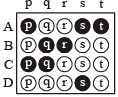Q.1. Match gases under specified conditions listed in Column I with their properties/laws in Column II. Indicate your answer by darkening the appropriate bubbles of the 4 × 4 matrix given in the ORS.

Column I                                        Column II

(A) Explosive                                      (p) NaN3

(B) Artificial gem                                (q) Fe3O4

(C) Self reduction                               (r) Cu

(D) Magnetic material                        (s) Al2O3

(t) Pb(N3)2

(u) Fe2O3

(v) Cu

(w) SiC

Ans. (A)-t; (B)-s; (C)-v; (D)-u

Solution. (A)-(t),   Pb(N3)2 is an explosive
(B)-(s),  Al2O3 is used to prepare artificial gun
(C)-(v),  Extraction of copper involves self-reduction process.
(D)-(u),  Fe2O3 is a magnetic material.

Q.2. Match the following :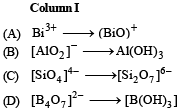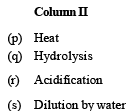Ans. (A)-q; (B)-s; (C)-p; (D)-r

Solution. (A)-(q), Bi3+ hydrolyses to yield BiO+ ion
(B)-(s), AlO2 on dilution yields a white ppt. of Al(OH)3
(C)-(p), When heated (SiO4)4– changes to (Si2O7)6–
(D)-(r), When acidified (B4O7)2– gives B(OH)3 (or H3BO3)

Q.3. Match each of the diatomic molecules in Column I with its property/properties in Column II.

Column I                                              Column II

(A) B2                                      (p) Paramagnetic

(B) N2                                     (q) Undergoes oxidation

(C) O2                                  (r) Undergoes reduction

(D) O2                                    (s) Bond order > 2

(t) Mixing of  's' and 'p' orbital

Ans. (A)-p, r, t; (B)-s, t ; (C)-p, q, r ; (D)-p, r, s

Solution.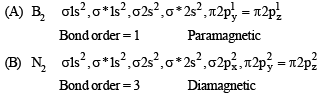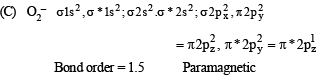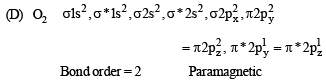DIRECTIONS (for Q. 4) : Following question has matching lists. The codes for the lists have choices (a), (b), (c) and (d) out of which ONLY ONE is correct.

Q.4. The unbalanced chemical reactions given in List I show missing reagent or condition (?) which are provided in List II. Match List I with List II and select the correct answer using the code given below the lists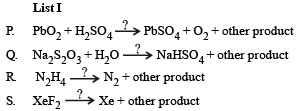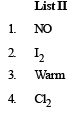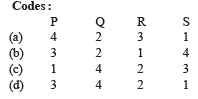Ans. (d)

Solution.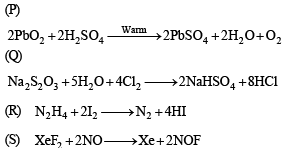Integer Value Correct Type

Q.1. The coordination number of Al in the crystalline state of AlCl3 is                  (2009)

Ans. 6

Solution. Coordination number of Al is 6. It exists in ccp lattice with 6 coordinate layer structure.

Q.2. The value of n in the molecular formula BenAl2Si6O18 is              (2010)

Ans. 3

Solution. Total cationic charge = Total anionic charge

2n + 6 + 24 = 36 ⇒ n = 3

Q.3. Reaction of Br2 with Na2CO3 in aqueous solution gives sodium bromide and sodium bromate with evolution of CO2 gas. The number of sodium bromide molecules involved in the balanced chemical equation is           (2011)

Ans. 5

Solution. 3Br2 + 3Na2CO 3 → 5NaBr + NaBrO3+ 3CO2

Q.4. Among the following, the number of compounds than can react with PCl5 to give POCl3 is  (2011)

O2, CO2, SO2, H2O, H2SO4, P4O10

Ans. 4

Solution. PCl5 + SO2 → POCl3 + SOCl2
PCl5 + H2O →POCl3 + 2HCl
PCl5 + H2SO4 → POCl3 + SO2Cl2 + 2HCl
6PCl5 + P4O10 → 10POCl3

Q.5. The total number of lone pairs of electrons in N2O3 is                (JEE Adv. 2015)

Ans. 8

Solution.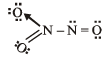Number of lone pairs = 8

Q.6. Three moles of B2H6 are completely reacted with methanol. The number of moles of boron containing product formed is    (JEE Adv. 2015)

Ans. 6

Solution. 3B2H6 + 18CH3OH → 6B(OCH3)3 + 18H2

Offer running on EduRev: Apply code STAYHOME200 to get INR 200 off on our premium plan EduRev Infinity!

69 docs|36 tests

,

,

,

,

,

,

,

,

,

,

,

,

,

,

,

,

,

,

,

,

,

,

,

,

,

,

,

;